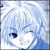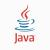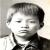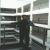各位大牛，调用类中private变量下的数组，编译时说是 [Linker error] undefined reference to 这个数组，是怎么回事啊？

【推荐阅读】滴滴出行的数据架构和信息流处理思路解析->>>#include<conio.h>
#include<fstream>
#include<iostream>
using namespace std;
class Vigenere
{
public:
Vigenere();
~Vigenere();
void Encrypt();//加密函数 ，将里面的英文字符进行加密
void Decipher();//解密函数
private:
static int key;

};
Vigenere::Vigenere()//构造函数
{
int key = {19,7,8,13,10};//密钥
}
Vigenere::~Vigenere()//析构函数
{
}
void Vigenere::Encrypt()
{

fstream infile,outfile;
infile.open("a.txt",ios::in);//读取明文文件
if(!infile)
{
cout<<"无法打开明文文件a.txt"<<endl;
abort();
}
outfile.open("b.txt",ios::out);
if(!outfile)
{
cout<<"无法打开密文文件b.txt"<<endl;
abort();
}
char ch;//用来存放从文件里取出的字符
int temp;//用来存放从文件里取出的字符转化为的数，(a或A对应数字0，b或B对应数字1，……)
int i = 0;//用来标记数组k[i]的下标
while(infile.get(ch))
{
if(ch >= 'A'&&ch <= 'Z')
{
temp = (int)ch - 65;//将字母转化为对应的数字 ，(a或A对应数字0，b或B对应数字1，……)
temp = (temp * 13 + key[i]) % 26 + 65;//加密算法为先乘以13，在加上key[i]的值，然后模26，加65是将其转换为字母所对应的字符
ch = (char)temp;//转换为字符
outfile.put(ch);//将加密后的字符赋值到目标文件里
i = (i + 1)%5;//使k下标在0到4之间循环
}else if(ch >= 'a'&&ch <= 'z')
{
temp = (int)ch - 97;
temp = (temp * 13 + key[i]) % 26 + 97;
ch = (char)temp;
outfile.put(ch);
i = (i + 1)%5;
}else//如果不是英文字符的话
outfile.put(ch);//保持源字符不变
}
infile.close();//关闭源文件
outfile.close();//关闭目标文件
}
void Vigenere::Decipher()//解密
{
fstream infile,outfile;
infile.open("b.txt",ios::in);
if(!infile)
{
cout<<"无法打开密文文件b.txt"<<endl;
abort();
}
outfile.open("c.txt",ios::out);
if(!outfile)
{
cout<<"无法打开明文文件c.txt"<<endl;
abort();
}
char ch;//用来存放从文件里取出的字符
int temp;//用来存放从文件里取出的字符转化为的数，(a或A对应数字0，b或B对应数字1，……)
int j = 0;//用来标记数组key[j]的下标
int n = 1;//用来标记所乘的13的倍数
while(infile.get(ch))
{
if(ch >= 'A'&&ch <= 'Z')
{
temp = (int)ch - 65;//将字母转化为对应的数字 ，(a或A对应数字0，b或B对应数字1，……)
//解密
temp = temp - key[j] + 26;
//加密是按照原数乘以13在加上key[i],然后模26的到的
//解密的话是先减去key[j]再加上26（在加上26的目的是为了防止出现负数）
while(temp % 13 != 0)
{
temp = temp + n * 26;
n++;
}
temp = temp / 13;
// 再加上n倍的26，最后然后除13取余看是否能够除尽，能除尽的最小数就是所要求的数
ch = (char)temp;//转换为字符
outfile.put(ch);//将解密密后的字符赋值到目标文件里
j = (j + 1)%5;//使key下标在0到4之间循环
}else if(ch >= 'a'&&ch <= 'z')
{
temp = (int)ch - 65;//将字母转化为对应的数字 ，(a或A对应数字0，b或B对应数字1，……)
//解密
temp = temp - key[j] + 26;
//加密是按照原数乘以13在加上key[i],然后模26的到的
//解密的话是先减去key[j]再加上26（在加上26的目的是为了防止出现负数）
while(temp % 13 != 0)
{
temp = temp + n * 26;
n++;
}
temp = temp / 13;
// 再加上n倍的26，最后然后除13取余看是否能够除尽，能除尽的最小数就是所要求的数
ch = (char)temp;//转换为字符
outfile.put(ch);//将解密密后的字符赋值到目标文件里
j = (j + 1)%5;//使key[j]下标在0到4之间循环

}else//如果不是英文字符的话
outfile.put(ch);//保持源字符不变
}
infile.close();//关闭源文件
outfile.close();//关闭目标文件
}
int main()
{
Vigenere vigenere;
vigenere.Encrypt();//加密
vigenere.Decipher();//解密
system("pause");
return 0;
}

[Linker error] undefined reference to `Vigenere::key'

0Vigenere::Vigenere()//构造函数

int key = {19,7,8,13,10};//密钥

key已经在上面定义过了，你在这里定义并赋值没有任何作用，因为构造函数执行完毕这几个就销毁了

0引用来自“leo108”的答案

Vigenere::Vigenere()//构造函数

int key = {19,7,8,13,10};//密钥

key已经在上面定义过了，你在这里定义并赋值没有任何作用，因为构造函数执行完毕这几个就销毁了

class Vigenere
{
public:
Vigenere();
~Vigenere();
void Encrypt();//加密函数 ，将里面的英文字符进行加密
void Decipher();//解密函数
private:
static int key;

};
int Vigenere::key = {19,7,8,13,10};
Vigenere::Vigenere()//构造函数
{
//int key = {19,7,8,13,10};//密钥
}
Vigenere::~Vigenere()//析构函数
{
}

0引用来自“leo108”的答案

Vigenere::Vigenere()//构造函数

int key = {19,7,8,13,10};//密钥

key已经在上面定义过了，你在这里定义并赋值没有任何作用，因为构造函数执行完毕这几个就销毁了

class Vigenere
{
public:
Vigenere();
~Vigenere();
void Encrypt();//加密函数 ，将里面的英文字符进行加密
void Decipher();//解密函数
private:
static int key;

};
int Vigenere::key = {19,7,8,13,10};
Vigenere::Vigenere()//构造函数
{
//int key = {19,7,8,13,10};//密钥
}
Vigenere::~Vigenere()//析构函数
{
}

Vigenere::Vigenere()//构造函数
{
key = 19;
key = 7;
key = 8;
key =13;
key = 10;
}
0引用来自“leo108”的答案

Vigenere::Vigenere()//构造函数

int key = {19,7,8,13,10};//密钥

key已经在上面定义过了，你在这里定义并赋值没有任何作用，因为构造函数执行完毕这几个就销毁了

class Vigenere
{
public:
Vigenere();
~Vigenere();
void Encrypt();//加密函数 ，将里面的英文字符进行加密
void Decipher();//解密函数
private:
static int key;

};
int Vigenere::key = {19,7,8,13,10};
Vigenere::Vigenere()//构造函数
{
//int key = {19,7,8,13,10};//密钥
}
Vigenere::~Vigenere()//析构函数
{
}

Vigenere::Vigenere()//构造函数
{
key = 19;
key = 7;
key = 8;
key =13;
key = 10;
}

class Vigenere

{

public:

Vigenere();

~Vigenere();

void Encrypt();//加密函数 ，将里面的英文字符进行加密

void Decipher();//解密函数

private:

int key;

};

//int Vigenere::key = {19,7,8,13,10};

Vigenere::Vigenere()//构造函数

{

//int key = {19,7,8,13,10};//密钥

key = 19;

key = 7;

key = 8;

key =13;

key = 10;

}

class Vigenere

{

public:

Vigenere();

~Vigenere();

void Encrypt();//加密函数 ，将里面的英文字符进行加密

void Decipher();//解密函数

private:

static int key;

};

//int Vigenere::key = {19,7,8,13,10};

Vigenere::Vigenere()//构造函数

{

//int key = {19,7,8,13,10};//密钥

key = 19;

key = 7;

key = 8;

key =13;

key = 10;

}

0引用来自“leo108”的答案

Vigenere::Vigenere()//构造函数

int key = {19,7,8,13,10};//密钥

key已经在上面定义过了，你在这里定义并赋值没有任何作用，因为构造函数执行完毕这几个就销毁了

0引用来自“leo108”的答案

Vigenere::Vigenere()//构造函数

int key = {19,7,8,13,10};//密钥

key已经在上面定义过了，你在这里定义并赋值没有任何作用，因为构造函数执行完毕这几个就销毁了

class Vigenere
{
public:
Vigenere();
~Vigenere();
void Encrypt();//加密函数 ，将里面的英文字符进行加密
void Decipher();//解密函数
private:
static int key;

};
int Vigenere::key = {19,7,8,13,10};
Vigenere::Vigenere()//构造函数
{
//int key = {19,7,8,13,10};//密钥
}
Vigenere::~Vigenere()//析构函数
{
}

0